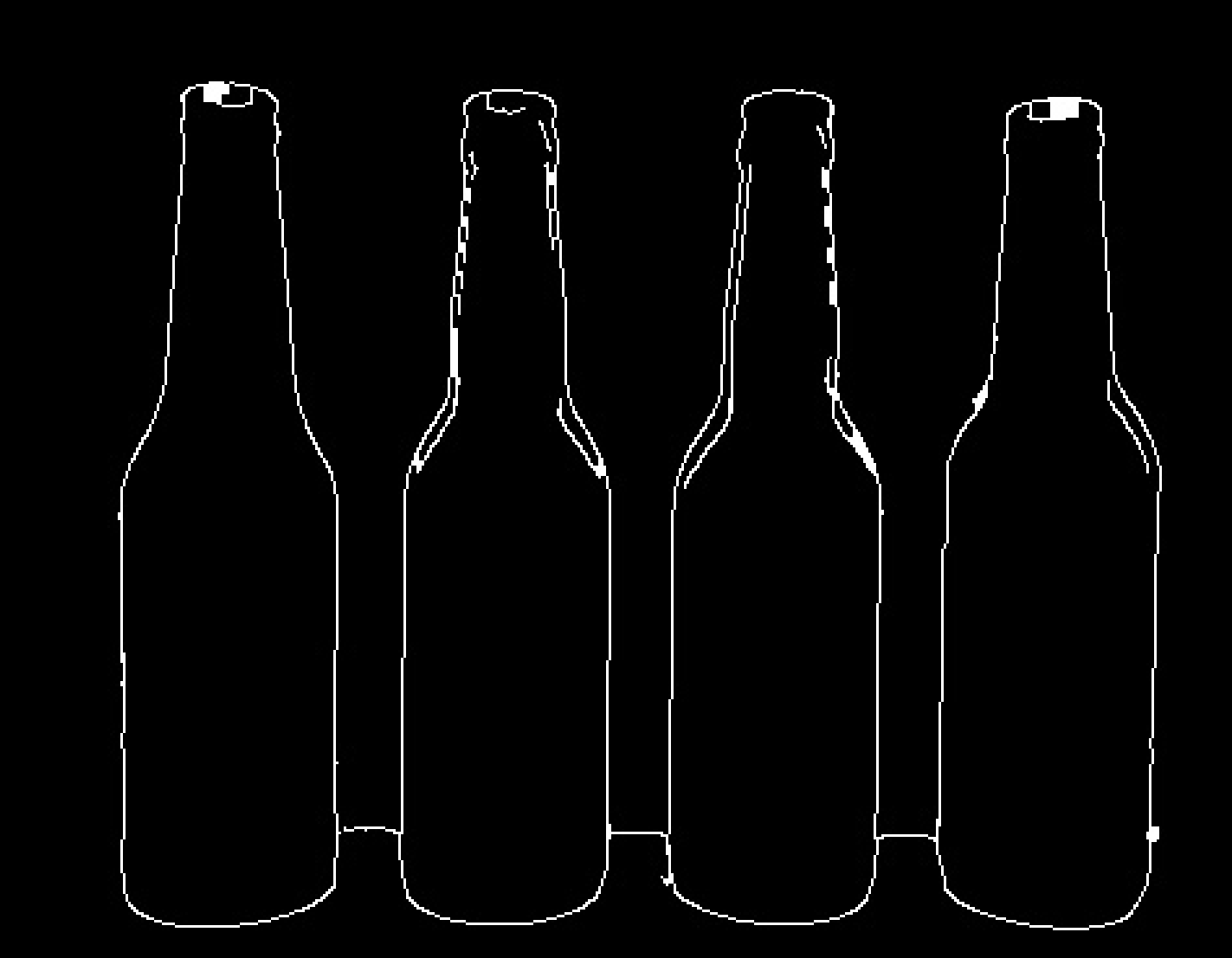# Process image to get rid of line between two components

Hello guys, currently i work on a project that uses image processing to crop out multiple bottles out of one image. In some of the images, the bottles are connected by a line (it is the edge of the table).

Sample image:Does anyone have a idea how i could get rid of this horizontal line between each bottle? My goal is to get only get the outline of each bottle.

I am thankful for any help :)

edit retag close merge delete

Sort by » oldest newest most voted

If I do an open morph operation with MorphologyEx using a rect kernel that is 12 x 1, I get this imageIf I subtract this from the original I getwhich is close. Before subtracting, you could find all the contours in the morphed image and filter to only include the table edge by looking at the y of the contour in the image and maybe the aspect ratio of the contour. After filtering, you could then dilate the remaining contours a little before subtracting.

more

Thank you a lot for your help ! i got it working now :)I had same @Chris's image. W/out using MorphologyEx and substraction. But never used it. Here is code:

#!/usr/bin/env python3
#OpenCV 4.0.1
#Date: 20th April, 2019

import cv2
import numpy as np

gray = cv2.cvtColor(img,cv2.COLOR_BGR2GRAY)
res = cv2.resize(gray, (640, 480))

horizontalStructure = cv2.getStructuringElement(cv2.MORPH_RECT, (1,2))
horizontal = cv2.erode(horizontal, horizontalStructure,  (-1, -1))
horizontal = cv2.dilate(horizontal, horizontalStructure,  (-1, -1))

numpy_horizontal = np.hstack((res, horizontal))
numpy_horizontal_concat = np.concatenate((res, horizontal), axis=1)

cv2.imshow("bottles", numpy_horizontal_concat)
cv2.imwrite("bottles2.jpg", numpy_horizontal_concat)
cv2.waitKey(0)
cv2.destroyAllWindows()


Output:horizontalStructure = cv2.getStructuringElement(cv2.MORPH_RECT, (1,3))


Output;more

The first image bottle 1 is not straight line. The rest are straight lines

thanks you so much ! i got it working now :)

Official site

GitHub

Wiki

Documentation